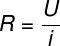Electricity & Megnetism

# Ammeter

Ammeters are electrical measuring instruments that are used to determine the intensity of electrical current flowing through a wire or electrical circuit.

The ammeter is a measuring device used to determine the intensity of electric current flowing through circuits and conducting wires. Ammeters can be digital or analog, however, inside, all ammeters are very similar, as they are built from an even more fundamental device – the galvanometer.

## What is the ammeter used for?

An ammeter is a device used to measure electrical current . For this, it is necessary to use it correctly and safely, respecting the necessary safety standards for its operation.

The symbol representing the ammeter is a simple circle that may or may not be accompanied by an arrow and the letter A in its center:

The symbol used to designate an ammeter.

## ideal ammeter

In the study of electricity, it is common to make the division between ideal and real ammeters. The ideal ammeter is one that can be inserted into electrical circuits without any interference . in its operation: the electrical current and voltage in the circuit do not undergo any change.

Ideal ammeters do not have electrical resistance, that is, they do not have the ability to oppose electrical current, and must be connected in series to the circuit, in this way, the electrical current can pass through it without suffering any potential drop.

All ammeters must be connected in series to the circuit.

Ammeters must be connected in series to the circuit.

## real ammeter

The real ammeter is the one used in everyday life. It is not perfect : it has an electrical resistance, even if very low, that is, this ammeter has a small capacity to oppose the electrical current. Therefore, when connecting it to the circuit, it is necessary to make sure that its resistance is much smaller than the resistance of the circuit, so that the measurements taken are not altered by the presence of the ammeter.

The clamp meter measures the electrical current without having to cut the wire.

## Formulas for ammeters

Most exercises involve installing ideal ammeters in electrical circuits made up of generators and resistors. In this case, to determine the electric current that is registered by these meters, it is necessary to calculate the equivalent resistance of the circuit, and also to use Ohm’s 1st Law , whose formula is shown below:U – Electric voltage
R – Electric resistance
i – Electric current

Also, remember the principles of electrodynamics , defined through Kirchoff’s laws , also known as the conservation of charge principle and the conservation of energy principle:

• Conservation of charges: The sum of all electrical currents reaching a circuit node is equal to the sum of electrical currents leaving it.
• Conservation of energy: In a circuit loop, the sum of all electrical potentials must be zero.

## electrical mters

In addition to ammeters, there are several devices that are used to measure electrical quantities. Check out the main electrical measurement devices and their main function:

• Multimeter : These are the most used electrical measuring devices, it has several functions, such as ammeter, voltmeter and ohmmeter.
• Voltmeter: It is used to measure the electrical voltage between two points in a circuit.
• Ohmmeter: This device is used to measure the electrical resistance of a wire or resistor.